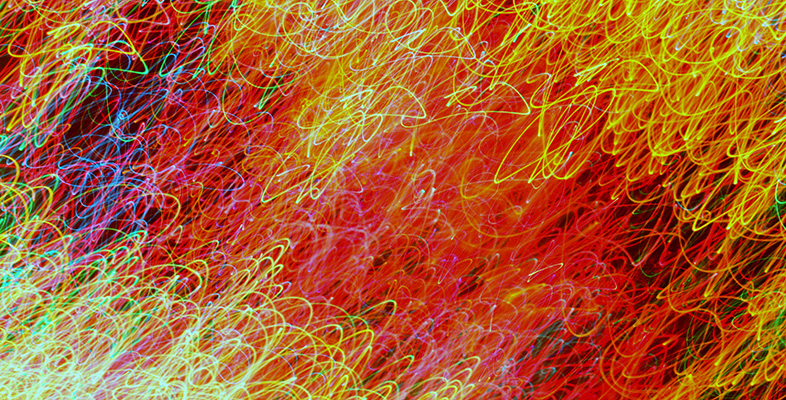Understanding the environment: Complexity and chaos

Start this free course now. Just create an account and sign in. Enrol and complete the course for a free statement of participation or digital badge if available.

# 3 Section activities

## Activity 5A: Exploring positive and negative feedback through system dynamics modelling

In this introductory activity the aim is to explore several simple system dynamics models as a means to visualise dynamic flows within a situation. What I love about system dynamics is that it uses a simple metaphor to capture how flows of energy, matter and information actually ‘work’ in reality. This metaphor is that of a bath with a pipe bringing water into it, and another pipe taking water out of it (Figure 5.2). The ‘system component’ is the bath, but what is important is what is in the bath, the ‘water level’, and how this changes over time.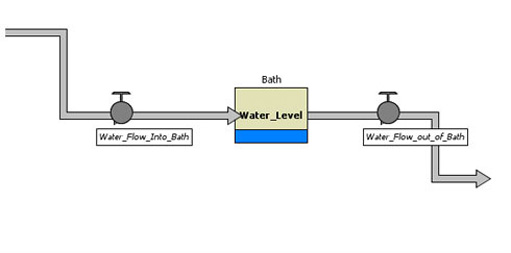Figure 5.2 System dynamics diagram using the ‘bath’ metaphor, showing ‘water level’ (the stock) and ‘water flow into bath’/‘water flow out of bath’ (the flows).

Modelling systems using system dynamics conventions is a good compromise between our need to understand reality as a collection of components and the actual flows of energy, matter and information that maintain, or modify, these components. System dynamics explicitly differentiates between ‘stocks’ (the baths) and ‘flows’ (the tap symbols determine the rate of flows in the pipes running into and out of the baths) that affect the levels of energy, matter and/or information temporarily ‘captured’ by the stocks. Human population number and global oil reserves are example of ‘stocks’, while birth rates and fuel extraction rates are example of ‘flows’. This is a useful differentiation since it is the change, over time, of the ‘stock levels’ as determined by the magnitude of the flow rates, which provides us with the indicators for understanding the dynamics of a system.

Once you begin to see systems in terms of stocks and flows, then it becomes impossible to look at them without noticing the tremendous movement of energy, matter and information that maintains apparently static states. Take the human body as an example – an apparently stable object is in fact only kept as such by a continuous exchange of flows of matter and energy with the environment, but stop those flows and viability is threatened. If you don’t believe me, see how long you can hold your breath!

In this initial activity, you will be introduced to several key relationships between stocks and flows which determine system dynamics. This introduction will lay the foundations for a more in-depth exploration of system dynamics and the NetLogo software.

### Activity 1

Start this activity by opening the Activity_5A_1.nlogo model. Resize and arrange the two distinct windows that appear (the System Dynamics Modeler window and the main NetLogo interface) so that you can see them both at the same time. (The layout of Figure 5.3 is just to allow convenient display on this web page.)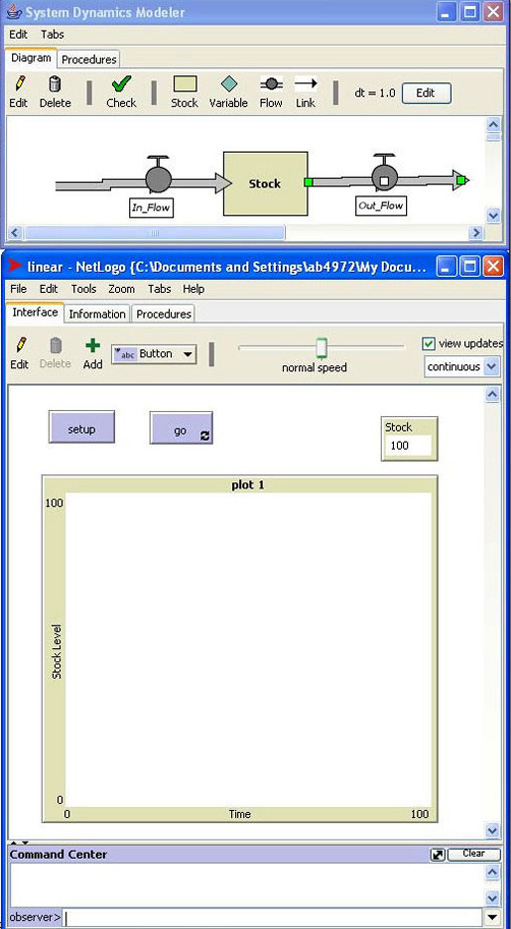Figure 5.3 Activity_5A_1.nlogo system dynamics model in NetLogo

On the System Dynamics Modeler window there are two tabs: ‘Diagram’ and ‘Procedures’. By default models open in the ‘Diagram’ tab, which allows you to view the model diagrammatically, whereas the Procedures tab gives a view of the mathematical procedures within the model. To meaningfully capture stocks and flows, system dynamics diagrams will usually contain the following four components:

• Stocks: representing the constituents of the model which will change in quantity over time.
• Rates: representing the ’taps’ which control the flows through which Stocks increase or decrease.
• Variables: representing additional values used in the flow rate calculations, which for convenience have not been (or cannot be) represented as stocks. These can include constants (values that do not change during the simulation) or equations which combine values from other Stocks or Variables. In this first model we have no constants – however you will encounter these very soon.
• Links: connecting Stocks and/or Variables to other Variables or Rates so that values from Stocks and/or Variables can be used in flow rate equations. Crucially, it is with these ‘links’ that feedback relationships are established.

If you now go to the System Dynamics Modeler window and double-click on the ‘In_Flow’ rate, you will see that it has a value of ‘2’, while double-clicking on the ‘Out_Flow’ rate reveals that it has a value of ‘1’. Double-clicking on the ‘Stock’ shows a value of ‘100’. The model simply means that for every iteration, 2 units will be added to the stock, and 1 unit will be detracted.

• i) What do you think will happen to the level of the ‘Stock’ over time?
• ii.What do you think will happen if you reverse the values for the ‘In_Flow’ and ‘Out_Flow’ rates?

Don’t run the simulation yet, but just note your answers on a piece of paper.

Now, open the Activity_5A_2.nlogo model. You should see the following model displayed in the System Dynamics Modeler (Figure 5.4).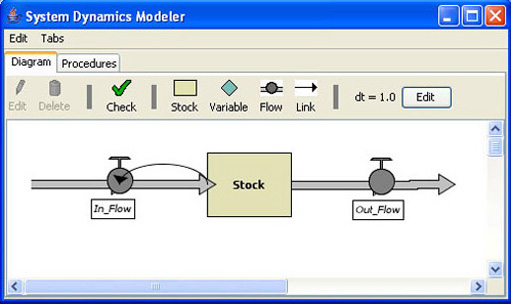Figure 5.4 Activity_5A_2.nlogo system dynamics model in NetLogo

Here the system dynamics diagram shows the ‘Stock’ linked back to the ‘In_Flow’ rate. By double-clicking on the ‘In_Flow’ tap you will see that in every iteration there is an increment to the stock level equivalent to the previous stock level. However, the ‘Out_Flow’ rate remains unchanged, with a value of ‘1’.

• i. What relationship do you think has been created between the ‘Stock’ and the ‘In_Flow’ rate?
• ii How do you think the ‘Stock’ level will change over time?

Once again, don’t run the simulation yet, but just note your answers.

Finally, open the last model for this activity, Activity_5A_3.nlogo. You should see the following model displayed in the System Dynamics Modeler (Figure 5.5).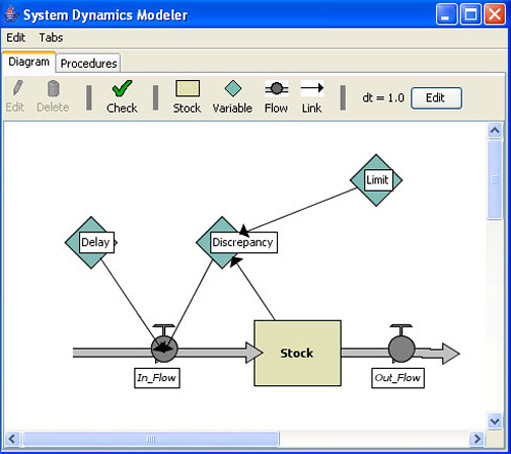Figure 5.5 Activity_5A_3.nlogo system dynamics model in NetLogo

If you double click on the ‘Discrepancy’ variable you will see that a simple formula subtracts the stock value from another value provided in the ‘Limit’ variable. This discrepancy then contributes towards the ‘In_Flow’ rate. In this case, the greater the difference between the ‘Stock’ and ‘Limit’ values, the greater the flows into the ‘Stock’. However, in order to slow down the rate of change, so that you can observe the dynamics more easily, I have also added a ‘Delay’ variable which is multiplied with the ‘Discrepancy’ value. In other words, the ‘In_Flow’ rate is determined by the 'limiting' effects of the 'Delay' variable on the 'Discrepancy' variable.

• i. What kind of dynamics do you think is established by this formulation?
• ii. How does it differ from the dynamics in the Activity_5A_1.nlogo and Activity_5A_2.nlogo models?

Once again, just note your results.

Now run all three models to see if they correspond to your predicted dynamics. In order to run the models go to the main NetLogo window and first click on the ‘setup’ button, and then on the ‘go’ button. You may wish to slow down the simulation by shifting the speed setting slider at the top of the window towards the left (you may get a better graph as a result). To stop the simulation, just click on the ‘go’ button again. My ‘answer’ below summarises some of the key points you should have picked up from this activity.

Figure 5.6 shows the results for the Activity_5A_1.nlogo simulation. You can clearly see that the change in ‘Stock’ levels is a linear one – each iteration adds one unit to the stock. This is a clear example of a system which has no positive and/or negative feedback relationships in place.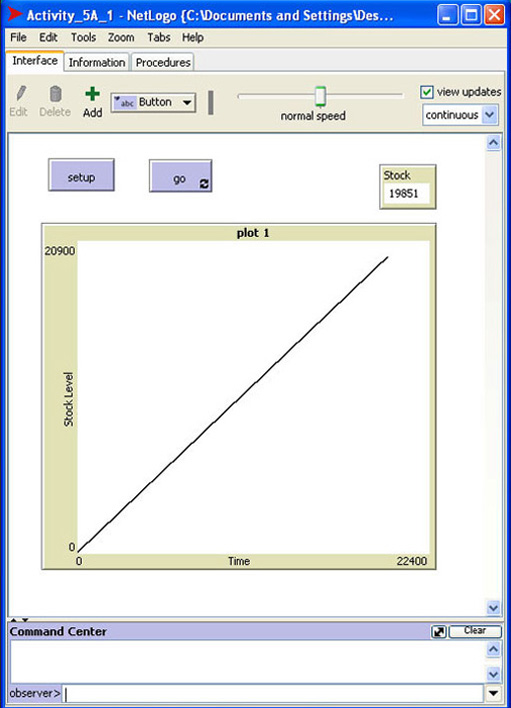Figure 5.6 Graph showing the results of the Activity_5A_1.nlogo model simulation.

Figure 5.7 shows the results for the Activity_5A_2.nlogo simulation. Here we have a positive feedback relationship between the ‘Stock’ and the ‘In_Flow’ rate: the larger the stock, the greater the ‘In_Flow’ rate. The exponential growth curve in the plot is a clear sign of a system dominated by one or more positive feedback relationships. The plot also shows why the term ‘non-linear’ is often used to explain the behaviour of complex systems. Although we won’t explore this idea further, it should be noted that for a dynamic system to be truly non-linear other relationships should also result in non-straight line graphs.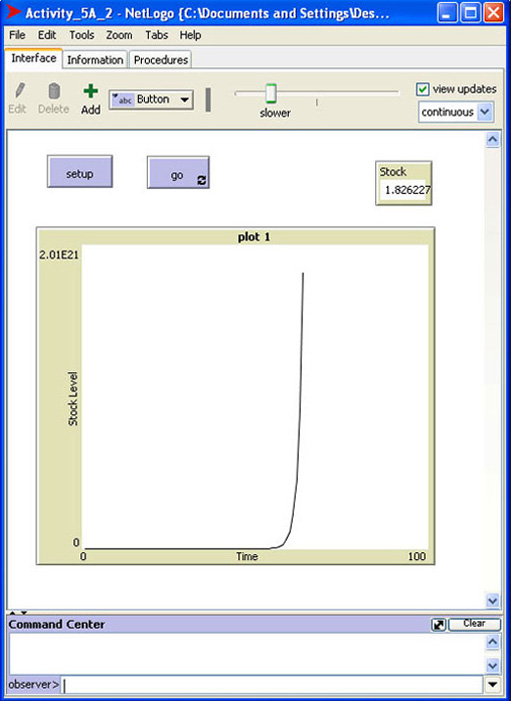Figure 5.7 Graph showing the results of the Activity_5A_2.nlogo model simulation.

Finally, Figure 5.8 shows the results for the Activity_5A_3.nlogo simulation. Here we have a negative feedback relationship between the ‘Stock’ and the ‘In_Flow’ rate as determined by the relationship established with the ‘Limit’ variable: the larger the ‘Stock’, the slower the ‘In_Flow’ rate. As the ‘Stock’ variable nears the value of the ‘Limit’ variable, then growth stops altogether. Living systems are kept in homeostasis thanks to simple relationships such as these.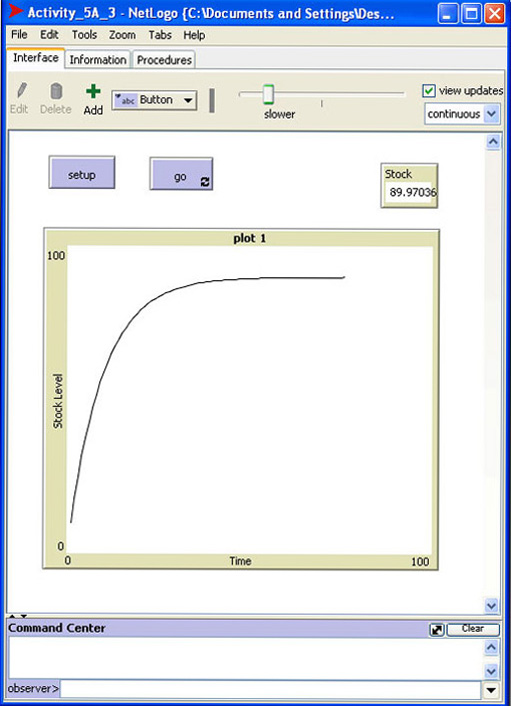Figure 5.8 Graph showing the results of the Activity_5A_3.nlogo model simulation.

One final point I would like to make about this activity is that, although I often talk about a particular ‘system dynamics diagram’, it is actually impossible to predict a priori whether a relationship will result in positive or negative feedback unless you look into how these relationships are processed by the rates. That is why system dynamics diagrams cannot stand alone and have to be presented with verbal and, preferably, mathematical descriptions. A graph of the resulting dynamic behaviour is also a key element of the system dynamics approach.